# Python Program to Convert Decimal to Hexadecimal

In this article, we've created some programs in Python, to convert a decimal number entered by user at run-time to its equivalent hexadecimal value. Here are the list of programs:

• Decimal to Hexadecimal using while loop and list
• Using int() and hex() methods
• Using user-defined function
• Using class
• Shortest Python code for decimal to hexadecimal conversion

Note - Before creating these programs, if you're not aware about steps used for the conversion, then refer to Decimal to Hexadecimal Conversion Steps and Example to get every required things.

## Decimal to Hexadecimal using while Loop and List

To convert decimal to hexadecimal number in Python, you have to ask from user to enter a number in decimal number system, then convert it into its equivalent hexadecimal value as shown in the program given below:

```print("Enter the Decimal Number: ")
decnum = int(input())

i = 0
hexdecnum = []
while decnum!=0:
rem = decnum % 16
if rem<10:
rem = rem+48
else:
rem = rem+55
rem = chr(rem)
hexdecnum.insert(i, rem)
i = i+1
decnum = int(decnum / 16)

i = i-1
while i>=0:
print(end=hexdecnum[i])
i = i-1
print()```

Here is its sample run: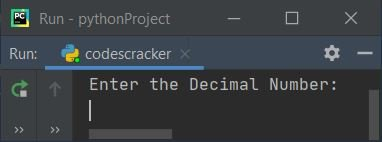Now supply the input say 50 as decimal number and press `ENTER` key to convert and print its equivalent value in hexadecimal as shown in the snapshot given below: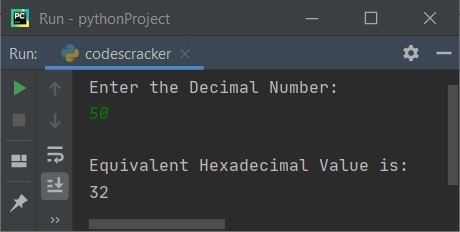Dry run of above program with user input 50 goes like:

• Initial values, decnum=50 (entered by user), i=0
• The condition (of while loop) decnum!=0 or 50!=0 evaluates to be true, therefore program flow goes inside the loop
• decnum%16 or 50%16 or 2 gets initialized to rem
• Now the condition (of if) rem<10 or 2<10 evaluates to be true, therefore program flow goes inside the if's body and rem+48 or 2+48 gets initialized to rem. Now rem=50. 50 is the ASCII of character 2
• Now chr(rem) or chr(50) or '2' gets initialized to rem. The chr() method is used to convert a value to a character type value.
• Now using the following statement:
`hexdecnum.insert(i, rem)`
the value of rem gets initialized to hexdecnum at ith index. That is, rem or 2 gets initialized to hexdecnum[i] or hexdecnum
• i+1 or 0+1 or 1 gets initialized to i
• int(decnum/16) or int(50/16) or 3 gets initialized to decnum
• Now program flow goes back and evaluates the condition of while loop again with new value of decnum. That is, decnum!=0 or 3!=0 evaluates to be true again, therefore program goes inside the loop. This process continues until the condition evaluates to be false
• In this way, we've converted decimal number entered by user to its equivalent hexadecimal value
• After exiting from the loop, print the element of list, hexdecnum[] from its last index.

#### Modified Version of Previous Program

This is the modified version of previous program. In this program, the end= is used to skip printing of an automatic newline using print().

```print("Enter the Decimal Number: ", end="")
dn = int(input())

i = 0
hdn = []

while dn!=0:
rem = dn % 16
if rem<10:
rem = rem+48
else:
rem = rem+55
hdn.insert(i, chr(rem))
i = i+1
dn = int(dn / 16)

print("\nEquivalent Hexadecimal Value = ", end="")
i = i-1
while i>=0:
print(end=hdn[i])
i = i-1

print()```

Here is its sample run with decimal number input as 107: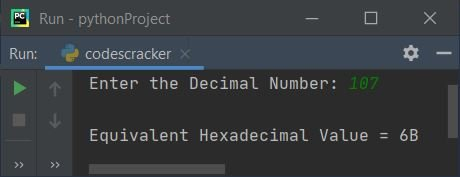## Decimal to Hexadecimal using int() and hex()

This program uses int() and hex(), predefined methods available in Python language to convert decimal to hexadecimal number.

```print("Enter Decimal Number: ", end="")
dn = input()

hdn = int(dn)
hdn = hex(hdn)

print("\nEquivalent Hexadecimal Value = ", hdn)```

Here is its sample run with user input, 3453 as decimal number:Note - To skip first two characters from hexadecimal output. And also capitalize all the hexadecimal digit, replace the following statement:

`print("\nEquivalent Hexadecimal Value = ", hdn)`

with the statement given below:

`print("\nEquivalent Hexadecimal Value = ", hdn[2:].upper())`

now the output after replacing the statement from above program, looks like: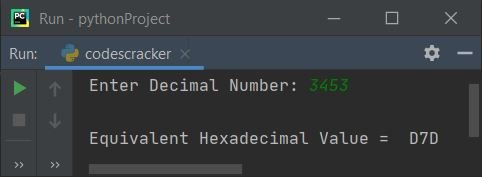While receiving inputs, by default, input type treated as a string type value. Therefore we've converted the entered value to an integer type value using int() and then using hex() method, the integer (decimal number) gets converted to a hexadecimal value.

Note - The hex() method returns equivalent hexadecimal value of a number passed as its argument.

## Decimal to Hexadecimal using Function

This program is created using a user-defined function named DecToHex(). The function takes a number as its argument and returns its equivalent hexadecimal value.

```def DecToHex(d):
h = int(d)
return hex(h)

print("Enter Decimal Number: ", end="")
dn = input()

hdn = DecToHex(dn)
print("\nEquivalent Hexadecimal Value = ", hdn[2:].upper())```

This program produces same output as of previous program.

## Decimal to Hexadecimal using Class

This is the last program created using class, an object-oriented feature of Python. The name of class in this program is CodesCracker

```class CodesCracker:
def DecToHex(self, d):
h = int(d)
return hex(h)

print("Enter Decimal Number: ", end="")
dn = input()

ob = CodesCracker()
hdn = ob.DecToHex(dn)
print("\nEquivalent Hexadecimal Value = ", hdn[2:].upper())```

Since to access any member function of a class in Python, we've to create and use class's object. Therefore an object named ob is created of class CodesCracker. And using this object, we've accessed the member function named DecToHex() of class CodesCracker, using dot (.) operator.

### Shortest Python Code for Decimal to Hexadecimal Conversion

This is the shortest code in Python, to convert decimal to hexadecimal number:

```dn = input()
print(hex(int(dn))[2:].upper())```

Here is its sample run with 234 as decimal number input: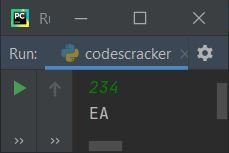#### Same Program in Other Languages

Python Online Test

« Previous Program Next Program »